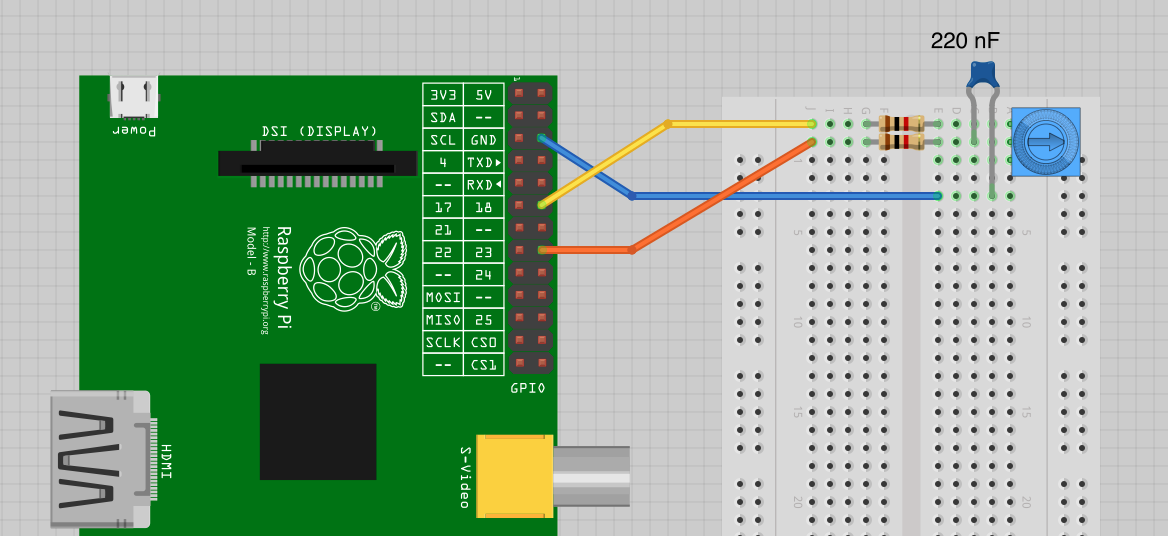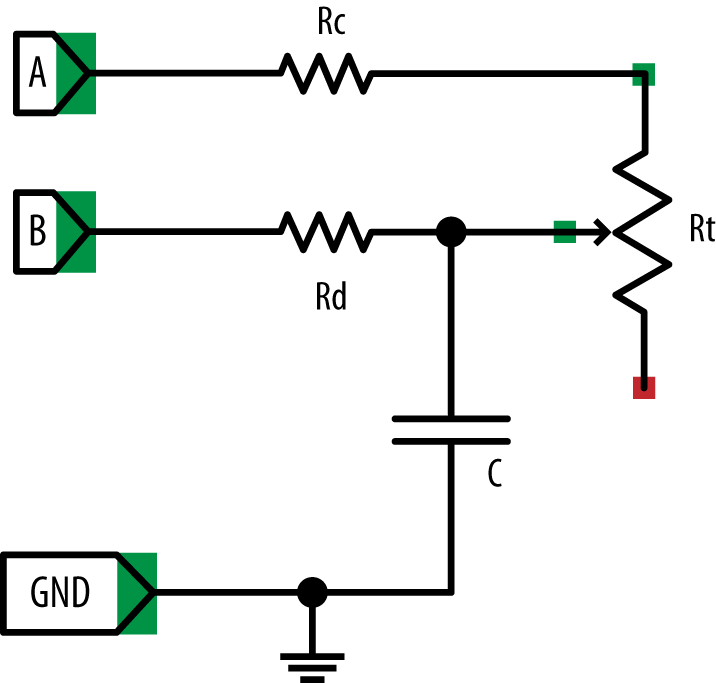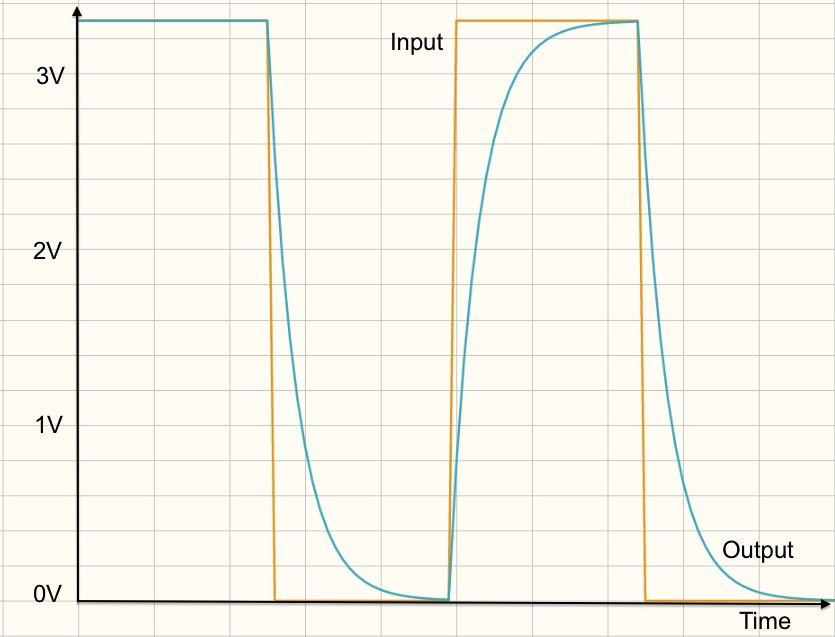# Using Resistive Sensors

## Problem

You want to connect a variable resistor to a Raspberry Pi and measure the position of its rotation.

## Solution

You can measure resistance on a Raspberry Pi using nothing more than a capacitor, a couple of resistors, and two GPIO pins. In this case, you will be able to read the position of the knob on a small variable resistor (trimpot).

To make this recipe, you will need:

Figure 8-1 shows the arrangement of components on the breadboard.Figure 8-1. Measuring resistance on a Raspberry Pi

Open an editor (nano or IDLE) and paste in the following code. As with all the program examples in this book, you can also download the program from the Code section of the Raspberry Pi Cookbook website, where it is called pot_step.py.

````import` `RPi.GPIO` `as` `GPIO`
`import` `time`

`GPIO``.``setmode``(``GPIO``.``BCM``)`

`a_pin` `=` `18`
`b_pin` `=` `23`

`def` `discharge``():`
`GPIO``.``setup``(``a_pin``,` `GPIO``.``IN``)`
`GPIO``.``setup``(``b_pin``,` `GPIO``.``OUT``)`
`GPIO``.``output``(``b_pin``,` `False``)`
`time``.``sleep``(``0.005``)`

`def` `charge_time``():`
`GPIO``.``setup``(``b_pin``,` `GPIO``.``IN``)`
`GPIO``.``setup``(``a_pin``,` `GPIO``.``OUT``)`
`count` `=` `0`
`GPIO``.``output``(``a_pin``,` `True``)`
`while` `not` `GPIO``.``input``(``b_pin``):`
`count` `=` `count` `+` `1`
`return` `count`

`def` `analog_read``():`
`discharge``()`
`return` `charge_time``()`

`while` `True``:`
`print``(``analog_read``())`
`time``.``sleep``(``1``)````

When you run the program, you should see some output like this:

```\$ sudo python pot_step.py
10
12
10
10
16
23
43
53
67
72
86
105
123
143
170```

The reading will vary between about 10 and about 170 as you rotate the knob of the trimpot.

## Discussion

To explain how this program works, I first need to explain how the step response technique can be used to measure the resistance of the variable resistor.

Figure 8-2 shows the schematic diagram for the recipe.Figure 8-2. Measuring resistance using step response

This way of doing things is called step response because it works by seeing how the circuit responds from the step change when an output is switched from low to high.

You can think of a capacitor as a tank of electricity, and as it fills with charge, the voltage across it increases. You can’t measure that voltage directly, because the Raspberry Pi doesn’t have an ADC converter. However, you can time how long it takes for the capacitor to fill with charge to the extent that it gets above the 1.65V or so that constitutes a high digital input. The speed at which the capacitor fills with charge depends on the value of the variable resistor (Rt). The lower the resistance, the faster the capacitor fills with charge and the voltage rises.

To be able to get a good reading, you must also be able to empty the capacitor each time before you take a reading. In Figure 8-2, connection A is used to charge the capacitor through Rc and Rt, and connection B is used to discharge (empty) the capacitor through Rd. The resistors Rc and Rd are used to make sure that there is no way too much current can flow as the capacitor is charged and discharged.

The steps involved in taking a reading are first to discharge the capacitor through Rd and then to let it charge through Rc and Rt.

To discharge it, connection A (GPIO 18) is set to be an input, effectively disconnecting Rc and Rt from the circuit. Connection B (GPIO 23) is then set to be an output and low. This is held there for 5 milliseconds to empty the capacitor.

Now that the capacitor is empty, you can start to allow charge to flow into it by setting connection B to be an input (effectively disconnecting it) and then enabling connection A to be a high output at 3.3V. Capacitor C will now begin to charge through Rc and Rt. The `while` loop will then simply count as fast as possible until the voltage at connection B switches from being low to high at about 1.65V.

At that point, the counting stops and the count value is returned.

Figure 8-3 shows how a resistor and capacitor in this kind of arrangement charge and discharge as the voltage is toggled between high and low.Figure 8-3. Charging and discharging a capacitor

You can see that the voltage at the capacitor increases rapidly at first but then tails off as the capacitor becomes full. Fortunately, you are interested in the area of the curve up until the capacitor reaches about 1.65V, which is a fairly straight line, meaning that the time taken for the voltage across the capacitor to rise to this point is roughly proportional to the resistance of Rt and hence the position of the knob.

This approach is not hugely accurate, but it is very low cost and easy to use.

## See Also

Using a step response works well with all kinds of resistive sensors for light (“Measuring Light”) and even gas detection (“Detecting Methane”).

For more accurate measurements of the trimpot position, see “Measuring a Voltage”, where the pot is used with an ADC converter.